Categories

# GoHz Frequency Converter Calibration

High quality power supplies are essential in the R&D and production lines of electrical and electronic manufacturing companies. Frequency converter is a device to transform a certain frequency (e.g. 50Hz, 60Hz) & voltage AC power into another voltage and frequency power supply, to simulate any power system standards in the world. It's a common device being used for various industries, laboratories and export-oriented electronic manufacturing companies. Calibration is essential for frequency converter to output precise frequency, voltage and wattage, here GoHz will show you how to do calibration for a frequency converter.

1. Open the reverse side of GoHz frequency converter display, and find the keys as following picture shows.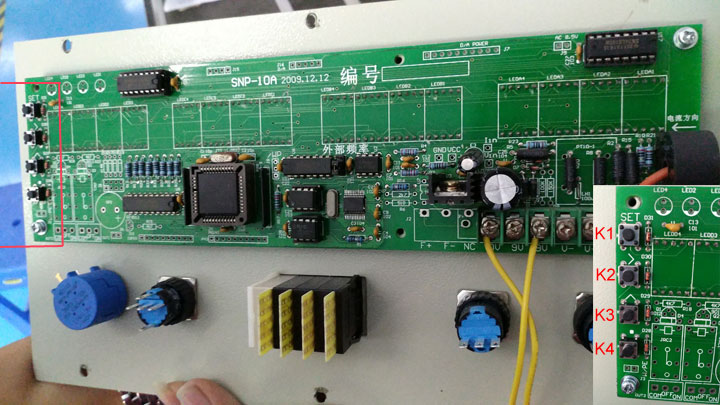Keys function:

1. K1 - SET key
2. K2 - Right Shift key
3. K3 - Increase key
4. K4 - Decimal key

2. Power on GoHz frequency converter, connect loads and turn on the output switch, press ON button (green button), then record the Voltage, Current, Wattage values on the converter's display.

3. Press K1 to set the password (Display will show as following picture).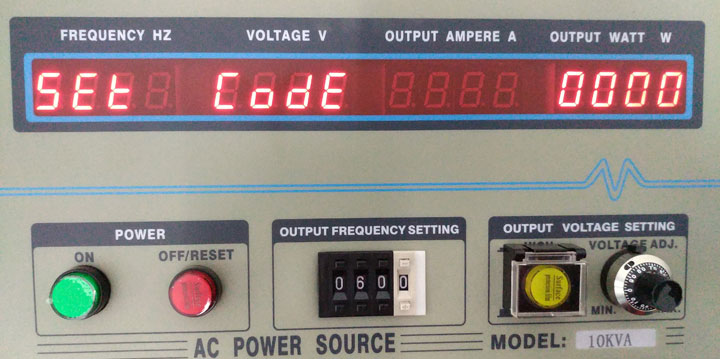4. Adjust K2 and K3 to enter the password "1234", shows as following picture, then press K1 to enter into the setting mode.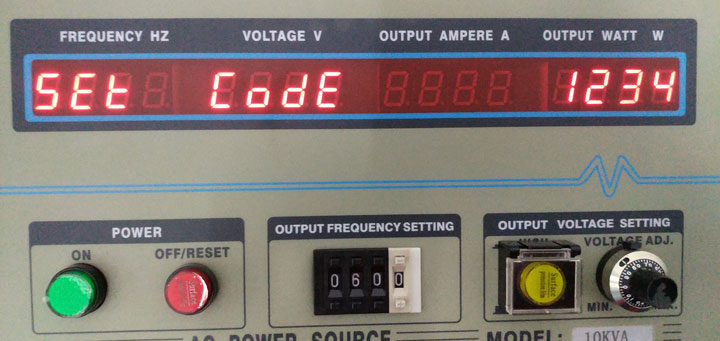5. Display shows as following picture means the calibration is Voltage, the value under the "OUTPUT WATT W" is the ratio of the Voltage. If the voltage on the display is less than the actual measured voltage, adjust K2 and K3 to set the ratio to a lower value (say 0.992); If the voltage on the display is larger than the actual measured voltage, adjust K2 and K3 to set the ratio to a larger value (say 1.005).6. Press K1 to enter into current setting, display shows as following picture means the calibration is Current, the value under the "OUTPUT WATT W" is the ratio of the Current. If the current on the display is less than the actual measured current, adjust K2 and K3 to set the ratio to a lower value (say 0.992); If the current on the display is larger than the actual measured current, adjust K2 and K3 to set the ratio to a larger value (say 1.005).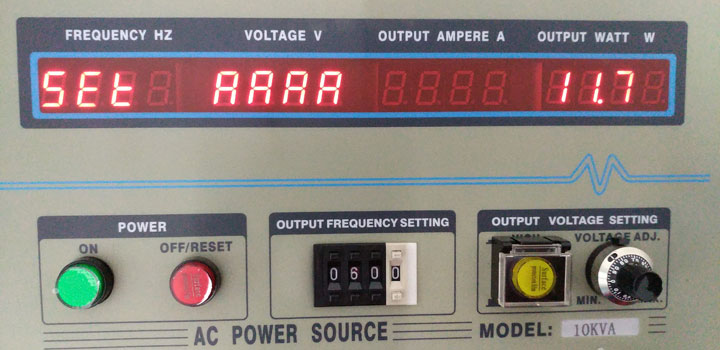7. Once the Voltage & Current calibration finished, press K1 repeatedly until the display shows as following picture, then press K2 to shift the blinking cursor to "Y" under the "OUTPUT WATT W", and press K1 to save the calibrated parameters and exit the setting mode.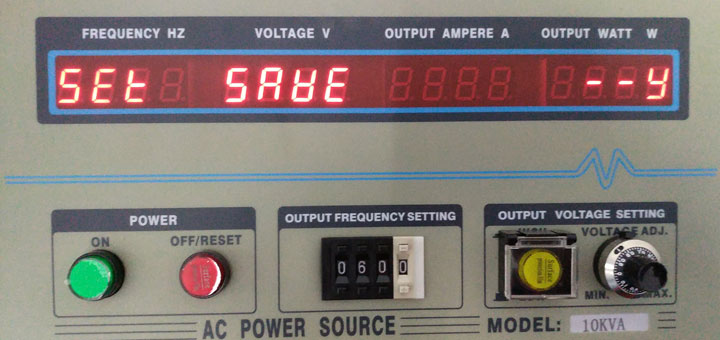8. Restart GoHz frequency converter and connect loads it, compare the Voltage & Ampere & Wattage values on the display with the actual measured values to see the deviations are eliminated or not, If not, repeat above calibrated steps to minimum the deviations.